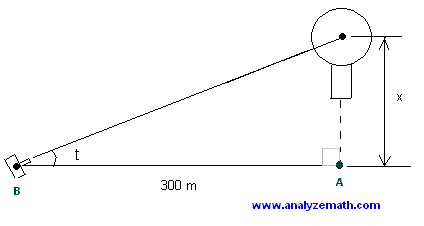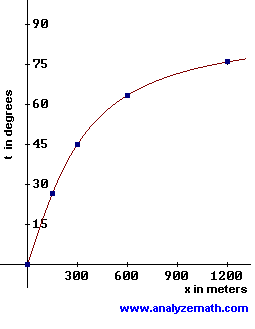# Applications and Use of the Inverse Functions

Examples on how to aplly and use inverse functions in real life situations and solve problems in mathematics.

## Example 1

Use inverse functions to solve equations.
Solve the following equation
Log ( x - 3) = 2

Solution to example 1
• Since logarithmic and exponential functions are inverses of each other, we can write the following.
A = Log (B) if and only B = 10 A
• Use the above property of logarithmic and exponential functions to rewite the given equation as follows.
x - 3 = 10 2
• Solve for x to obtain.
x = 103

## Example 2

Use inverse functions to find range of functions.
Find the range of function f give by
f(x) = 2 x / (x - 3)

Solution to example 2:
• We know that the range of a one to one function is the domain of its inverse. Let us first show that function f given above is a one to one function. Start with .
f(a) = f(b)
2 a / (a - 3) = 2 b / (b - 3)
• Multiply all terms of the above equation by (a - 3)(b - 3) and simplify to obtain.
2a (b - 3) = 2 b(a - 3)
• Expand.
2a b - 6 a = 2 ba - 6 b
• Add - 2 a b to both sides and simplify to obtain.
a = b
• Hence the given function is a one to one. let us find its inverse.
y = 2 x / (x - 3)
• Interchange x and y and solve for y.
x = 2 y / (y - 3)
y = 3x / (2 - x)
• The inverse of function f is given by.
f-1 (x) = 3x / (2 - x)
• The domain of f-1 is the set of all real values except x = 2. Hence the range of f is the set of all real values except 2.

## Example 3

Use inverse functions find the angle of elevation of a camera.
A camera is to take a series of photographs of a hot air balloon rising vertically. The distance between the camera at (B) and the launching point of the balloon (A) is 300 meters. The camera must keep the balloon on sight and therefore its angle of elevation t must change with the height x of the balloon.
a) Find angle t as a function of the height x.
b) Find angle t in degrees when x is equal to 150, 300 and 600 meters. (approximate your answer to 1 decimal place).
c) Graph t as a function of x.Solution to example 3
• a) The opposite and the adjacent sides to angle t are x and 300 meters respectively, hence.
tan(t) = x / 300
• We now use the property of the tangent function and its inverse.
tan -1(tan(x)) = x
• To rewrite the equation tan(t) = x / 300 as follows.
tan -1(tan(t)) = tan -1( x / 300 )
• Simplify the left side of the above equation to obtain.
t = tan -1( x / 300 )
• b) The values of t at 150, 300 and 600 are found using a calculator..
t(150) = 25.6 degrees (approximated to 1 decimal place)
t(300) = 45.0 degrees
t(600) = 63.4 degrees (approximated to 1 decimal place)
• c) We use the values of t in part b) and extra points and graph t as a function of x..
x t
0 0
150 25.6
300 45.0
600 63.4
1200 76.0
3000 84.3## Example 4

Use inverse functions to find radius of right circular cone.
Five right circular cones, with the same height h = 50 cm, are to be constructed. The volumes of these cones are to be 200, 400, 800, 1600 and 3200 cm
3. Find the radius of the base of each cone.
Solution to example 4
• The formula of the volume V of a right circular cone with height h and radius r is given by.
V = (1/3) pi r2 h
• Since we need to find the radius, we need to solve the above eqaution for r to obtain.
r = SQRT(3 V / pi h)
• What we have done above is to find the inverse function of V. We do not need to interchange the variables V and r because they have different meaning in this problem..
• We now calculate the radius for each cone using the formula for the radius above..
a) V = 200 , r = SQRT(3*200 / 50*pi) = 1.95 cm
b) V = 400 , r = SQRT(3*400 / 50*pi) = 2.76 cm
c) V = 800 , r = SQRT(3*800 / 50*pi) = 3.91 cm
d) V = 1600 , r = SQRT(3*1600 / 50*pi) = 5.53 cm
e) V = 3200 , r = SQRT(3*3200 / 50*pi) = 7.82 cm

## Example 5

Use inverse functions to solve population problems.
The population of a certain city increase according to the following formula
P = 200,000 e 0.01 t

where P is the population and t the number of years, with t = 0 corresponding to the year 2000.
When will the population be 300,000, 400,000 and 500,000?
Solution to example 5
• We need to find t first by solving the given formula for t. Divide both sides of the given formula by 200,000..
P / 200,000 = e 0.01 t
• We now use the fact that the exponential and logarithmic functions are inverses of each other and rewrite the above exponential expression as follows.
0.01 t = ln ( P / 200,000 )
• Solve for t..
t = ln ( P / 200,000 ) / 0.01
• We now find t for the different values of P given above..
a) P = 300,000 , t = ln ( 300,000 / 200,000 ) / 0.01 = 40.55 years , year 2041
a) P = 400,000 , t = ln ( 400,000 / 200,000 ) / 0.01 = 69.31 years , year 2070
a) P = 500,000 , t = ln ( 500,000 / 200,000 ) / 0.01 = 91.63 years , year 2092

## More References and Links to the Inverse Functions

Find the Inverse Function - Questions